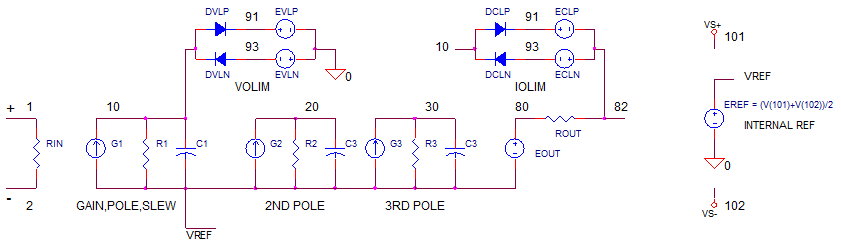## Op Amp Builder - Level 2

How can you create an intermediate SPICE model that includes slew-rate limiting and voltage/current output limits? Here's a JavaScript Calculator that shows you how! Add 2nd/3rd poles to capture the overshoot / ringing of a real op amp. Modify it any way you like, create your own models! Learn how JavaScript can create forms, SPICE models and print it to a text box.

Check out other JavaScript Calculators.

To learn to create these SPICE op amp models:
Quick intro to op amp models
Basic op amp model
Learn various op amp modelling techniques.

### SPICE Circuit### Enter Basic Behaviors

 Aol Open-Loop Gain (V/V) fu Unity-gain Frequency (Hz)or Gain Bandwidth Product (GBP) Slew Max Max Vo change per micro second (V/us) Rin Input R Rout Output R

### Enter 2nd and 3rd Poles

 fp2 2nd pole fp3 3rd pole Initially set fp2=fp3=10 x fu.Set to 100 x fu to remove effect.

### Enter Voltage and Current Limits

 Vo lim How close Vo swings to supply rails Io lim Max output current

### Enter Model Parameters

 Subckt Name Name for SPICE subcircuit. Device Name Name for SPICE subcircuit. Precision (digits) Precision for moldel values.

### Calculations

 C1 = I1max / (slew * 1000000) Capacitor for slew max and fp1. fp1 = fu / Aol First pole filter to acheive fu. R1 = 1 / (2 * pi * fp1 * C1) Resistor for fp1 and Aol. KG1 = Aol / R1 Open-Loop Gain Aol = KG1 * R1. C2 = 1 / (2 * pi * fp2 * R2) C for fp2. C3 = 1 / (2 * pi * fp3 * R3) C for fp3. EVL (See code) Voltage source for Vo Limit. ECL (See code) Voltage source for Io Limit.

To copy into text file, First Ctrl-A and Ctrl-C inside box above, then Ctrl-V into text file